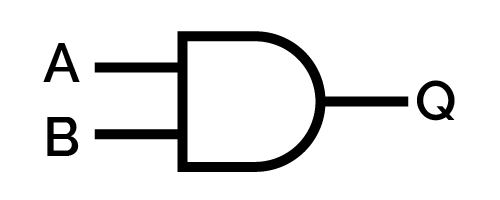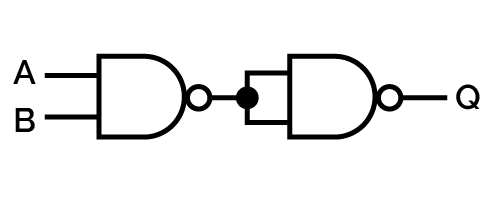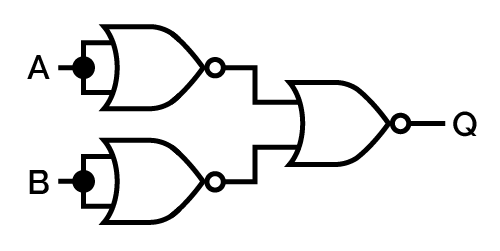# AND Calculator

Created by Davide Borchia
Reviewed by Anna Szczepanek, PhD and Adena Benn
Last updated: May 30, 2023

With our AND calculator, you will learn one of the most important logic operations. Keep reading this short but exhaustive article to learn:

• What is the AND logical operator;
• The logic AND gate: symbol, operations, relationship with other logic gates;
• How to calculate the AND in binary; and
• How to use our AND calculator.

What are you waiting for? Follow us!

## AND: the logical conjunction

In logic, AND has the same function as the word and in grammar (at least in some circumstances). It joins two or more elements, implying that they are all true in the same moment, space, or situation.

AND is a logical operator, something that resembles an arithmetic operator but that works only on a restricted set of values:

• True, or $1$;
• False, or $0$.

The logical AND operator returns true only if all the input operands are true. A single false input causes the result to become false, regardless of the accuracy of any other number of inputs.

We indicate the AND operator in many ways. Considering two operand ($A$, $B$), we can find the operator expressed:

• In a verbose fashion, using the word shared by natural language. In this case, we write $A\mathrm{\ AND\ }B$.

• In mathematics we can use the symbol $\land$, as in $A\land B$.

• In electronics, we use the simple centered dot instead: $A\cdot B$.

• In many programming languages, the logic AND operator is represented in condition by either $\&$ or $\&\&$. You may also find $\rm and$ in some languages.

• To conclude, in Łukasiewicz's logic, we use a prefix operator, $\mathrm{K}$. You can learn more about the work of this scientist with our polish notation converter.

## How to calculate the AND in binary

Here we will learn how to calculate the AND logic gate in binary. As it turns out, logic operations share many similarities with binary arithmetic. Calculating the logic AND between two binary numbers corresponds to performing a bitwise product — which means computing the product digit by digit, considering digits in matching positions. Each bit can have one of two values: $1$ or $0$, hence we can find four possible cases:

• $0\times0 = 0$;
• $0\times1=0$;
• $1\times0=0$; or
• $1\times1=1$.

Only the last operation returns a value different from $0$. Let's see an example of this operation!

Take two binary numbers, for example, $10101$ and $11001$: how do we perform the AND operation?

1. Stack the numbers one over the other, aligning them to the right.
2. Start taking pairs of bits in the same position.
3. Multiply them.
4. Build the result progressively.

In our case, we'd write:

$\begin{split} 10101&\land\\ 11001&= \end{split}$

We consider the first pair on the right: $1$, $1$: the result is $1\times 1 = 1$. Note this.

Next step, $0$, $0$: this is the opposite operation: $0\times 0=0$. The third position is our first mixed operation: $0\times 1=0$, etc. The result is:

$\begin{split} 10101&\land\\ 11001&=\\ \hline 10001 \end{split}$

You can easily calculate the AND logic operator for a higher number of operands: simply keep adding factors to the product. You can see how all it takes is for one of them to be $0$ to cause the entire product to collapse to $0$.

## The truth table of the AND logic gate

One last way to quickly remember the behavior of the AND logic gate is to memorize its truth table. A truth table is a minute table that contains exclusively the inputs of a logical operator alongside its result. For an AND gate, it would look like this:

$\text{\textbf{in}}_\bold{1}$

$\text{\textbf{in}}_\bold{2}$

$\text{\textbf{output}}$

$0$

$0$

$0$

$1$

$0$

$0$

$0$

$1$

$0$

$1$

$1$

$1$

In this case, too, you can add more columns, one for each additional operand. The truth table for the logical AND ternary operator is:

$\text{\textbf{in}}_\bold{1}$

$\text{\textbf{in}}_\bold{2}$

$\text{\textbf{in}}_\bold{3}$

$\text{\textbf{output}}$

$0$

$0$

$0$

$0$

$1$

$0$

$0$

$0$

$0$

$1$

$0$

$0$

$0$

$0$

$1$

$0$

$1$

$1$

$0$

$0$

$1$

$0$

$1$

$0$

$0$

$1$

$1$

$0$

$1$

$1$

$1$

$1$

## The logic AND gate

In logic circuitry, a branch of electronics that studies the construction of circuits that operate according to boolean logic (true/false logic), the logical AND gate is one of the most important elements (yet not the fundamental one: the crown goes to the NAND gate or the NOR gate, depending on the logic used).

🙋 Why do we call it AND gate? Because it takes something as input, filters it, eventually giving a specific result as output: the inputs must pass through it as much as we need to go through gates to enter a garden or a building.

The symbol for the AND gate in a logic circuit is the following:In the NAND logic, a paradigm for which all logic gates (OR, XOR, NOT, etc.) are represented using only NAND gates, the AND logic gate is simply the negation of the fundamental NAND gate. To negate the NAND gate, we can simply take the output of a NAND gate, duplicate it, then use it as input to a second NAND gate. The NAND that returns $1$ receives two $0$s, or vice-versa. To produce $0$, we feed it two $1$s, as opposed to the AND gate, that when provided two $1$s returns $1$, or which, when it receives two $0$s, returns $0$).Look at the following truth table to understand what we mean:

$\text{\textbf{A}}$

$\text{\textbf{B}}$

$\text{\textbf{out}}$

$\text{\textbf{A}}_{\boldsymbol{1}}$

$\text{\textbf{B}}_{\boldsymbol{1}}$

$\text{\textbf{Q}}$

$0$

$0$

$1$

$1$

$1$

$0$

$1$

$0$

$1$

$1$

$1$

$0$

$0$

$1$

$1$

$1$

$1$

$0$

$1$

$1$

$0$

$0$

$0$

$1$

In the similar NOR logic, the AND gate requires a more complex construction that involves three NOR gates. You can see the related circuit in the image below.If you are interested in the basis of NOR logic, visit our NOR calculator.

## How to use our AND calculator

Our AND calculator implements the bitwise AND operation. Hence it operates in the binary base. However, you are not limited to binary inputs. To use our logical AND calculator, follow these easy steps:

1. Select the binary representation you are interested in. This quantity controls the number of bits you can input into your number. For example, the 4-bit representation allows you to input numbers from $-2^4-1$ to $2^4$ (the slightly smaller range in the negative region is due to the one's complement. You can learn everything about it at our one's complement calculator).

2. Once you have restricted the input range, choose the numerical base you desire (binary, octal, decimal).

3. Input the two numbers of your interest: we will print the result of the logic AND calculations.

🙋 You can find a generic tool for more logic gates at the bitwise calculator. For specific insights on a similar gate, try our XOR calculator.

## FAQ

### What is the AND logic operator?

The AND logic operator is a logic operator corresponding to a conjunction, which means that to result in a true statement, all the inputs must be true. The AND logic operator corresponds to the binary bitwise product.

### How do I calculate numbers using the AND logic operator?

To calculate numbers using the AND logical operator:

1. Take the function's inputs.
2. If expressed as a series of true/false, convert it to binary with the simple rules true = 1, false = 0.
3. Take each pair of digits, bitwise.
4. Compute the product of each pair.
5. Write the results in the proper order.

You are done!

### What is 10100 AND 11011?

The result of the logic AND operation 10101∧11011 is 10001. To find this result, follow these easy steps:

1. Align the two numbers to the right.
2. Using digits in the same position, perform the product.
3. Be sure to note it in the results. For example, the first operation would be 1 × 1 = 1, the second one 0 × 1 = 0, etc.
4. Once you reach the last digit, you are done!

### Can the AND gate have three inputs?

Yes. The AND gate accepts more than two inputs. The truth table of a logic AND gate is quickly expanded to multiple inputs, as it only suffices to find all the possible combinations of inputs, keeping the last one with all trues as the only one resulting in a true in output. In terms of circuitry, associate every pair of inputs with the AND gate. The output will feed the next gate until there is a single output.

Davide Borchia
Binary representation
8-bit
Datatype
Decimal
You can enter decimal numbers from -128 to 127.
First number
Second number
People also viewed…

### Body fat

Use the body fat calculator to estimate what percentage of your body weight comprises of body fat.

### Percentage of a percentage

Percentage of percentage allows you to multiply % by % and get the result.